# ISEE Lower Level Quantitative : How to compare fractions

## Example Questions

### Example Question #2774 : Isee Lower Level (Grades 5 6) Quantitative Reasoning

Select the symbol to correctly fill in the blank below.__________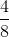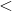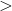Explanation:

To compare fractions, we need to first make common denominators.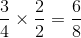Now that we have common denominators, we can compare numerators. The fraction with the bigger numerator has the greater value.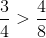### Example Question #2775 : Isee Lower Level (Grades 5 6) Quantitative Reasoning

Select the symbol to correctly fill in the blank below.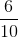__________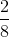Explanation:

To compare fractions, we need to first make common denominators.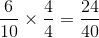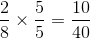Now that we have common denominators, we can compare numerators. The fraction with the bigger numerator has the greater value.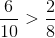### Example Question #2776 : Isee Lower Level (Grades 5 6) Quantitative Reasoning

Select the sign to correctly fill in the blank: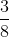__________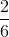Explanation:

To compare fractions, we need to first make common denominators.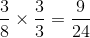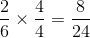Now that we have common denominators, we can compare numerators. The fraction with the bigger numerator has the greater value.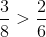### Example Question #2777 : Isee Lower Level (Grades 5 6) Quantitative Reasoning

Select the symbol to correctly fill in the blank below.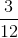__________Explanation:

To compare fractions, we need to first make common denominators.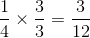Now that we have common denominators, we can compare numerators. The fraction with the bigger numerator has the greater value.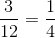### Example Question #2778 : Isee Lower Level (Grades 5 6) Quantitative Reasoning

Select the symbol to correctly fill in the blank below.__________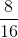Explanation:

To compare fractions, we need to first make common denominators.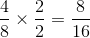Now that we have common denominators, we can compare numerators. The fraction with the bigger numerator has the greater value.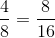### Example Question #2779 : Isee Lower Level (Grades 5 6) Quantitative Reasoning

Select the symbol to correctly fill in the blank below.__________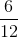Explanation:

To compare fractions, we need to first make common denominators.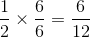Now that we have common denominators, we can compare numerators. The fraction with the bigger numerator has the greater value.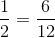### Example Question #2780 : Isee Lower Level (Grades 5 6) Quantitative Reasoning

Select the symbol to correctly fill in the blank below.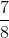__________Explanation:

To compare fractions, we need to first make common denominators.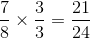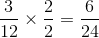Now that we have common denominators, we can compare numerators. The fraction with the bigger numerator has the greater value.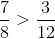### Example Question #2781 : Isee Lower Level (Grades 5 6) Quantitative Reasoning

Select the symbol to correctly fill in the blank below.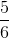__________Explanation:

To compare fractions, we need to first make common denominators.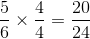Now that we have common denominators, we can compare numerators. The fraction with the bigger numerator has the greater value.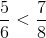### Example Question #2782 : Isee Lower Level (Grades 5 6) Quantitative Reasoning

Select the symbol to correctly fill in the blank below.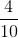__________Explanation:

To compare fractions, we need to first make common denominators.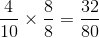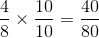Now that we have common denominators, we can compare numerators. The fraction with the bigger numerator has the greater value.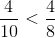### Example Question #2783 : Isee Lower Level (Grades 5 6) Quantitative Reasoning

Select the symbol to correctly fill in the blank below.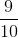__________Explanation:

To compare fractions, we need to first make common denominators.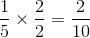Now that we have common denominators, we can compare numerators. The fraction with the bigger numerator has the greater value.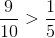### All ISEE Lower Level Quantitative Resources